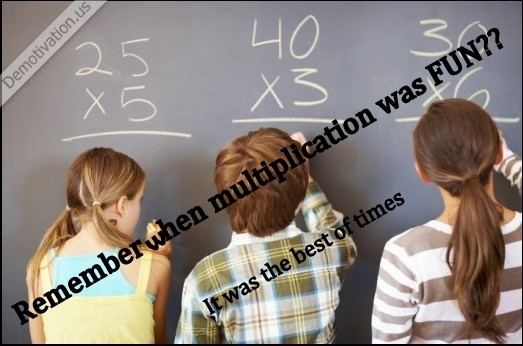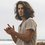# Have Fun Multiplying ![This contains original Research work conducted by ROMITH RAO. The Paper has been submitted to The Journal of The Ramanujan Mathematical Society. For more information www.Romithrao.com ]

For the first time ever a General formula for Multiplying any Two Numbers ( Integers). Yes! for the first Time in the world one formula that holds good for multiplying any two numbers from 1 to infinity!!

Let x and y be any two integers with integers u1 and u2 in their units place respectively. Then
x * y= [(x-u1) * (y-u2)] + {[(x-u1) * u2]+[(y-u2) * u1]} +u1u2 .

Multiplying any two integers in three simple steps:

Consider any two integers x and y with u1 and u2 being the numerals in their unit’s place respectively. Example 1: 348 * 85

Step1: multiply the numerals in the unit’s place. Now write the numeral in the unit’s place of the result in the unit’s place of the answer. Carry the remainder to the next step. 8 * 5 is 40. Write 0 in the unit’s place of the answer. Carry 4 to step 2

Step 2: Now ignore the numeral in the unit’s place and consider the new integers so formed which is 34 and 8. Now multiply these 2 integers from the numeral in the units’ place of the other integer and add them together. Add the remainder from the previous step to this. Now place the numeral in the unit’s place of this result in the ten’s place of the answer. Carry the remainder to the next step.

(34 * 5) + (8 * 8) +4 = 170+64+4= 238. Now place 8 in the ten’s place of the answer and carry 23 to step 3

Step 3: multiply the two newly formed digits i.e. 34 * 8= 272. Now add the remainder from the previous step. 272 +23 = 295 Answer :29580.

I. The general formula for the product of any two integers.

Derivation: (consider the above example)

Let 348 and 85 be x and y with 8 and 5 being u1 and u2 numerals in the units’ place respectively.

In this case 295 corresponds to 29500

That is 272 corresponds to 27200 and 23 corresponds to 2300

29580 = 27200 + 2340 + 40

Now 27200 = 340 * 80

2340 = (340 * 5) + (80 * 8)

40= 8 * 5

29580 = (340 * 80) + [(340 * 5) + (80 * 8) ] + 8 * 5

348 * 85 = [(348-8)(85-5)] +[(348-8 ) * 5+(85-5) * 8] +8 * 5 corresponds to

x * y= [(x-u1) * (y-u2)] + {[(x-u1)u2]+[(y-u2) * u1]} +u1u2 . This is the required general formula.

On simplification we get x * y = xy - u1y - xu2 + u1u2 + xu2 - u1u2 + yu1- u1u2 + u1u2
x * y = xy . Thus we have derived the general formula for the product of any two integers as:

x * y= [(x-u1)* (y-u2)] + {[(x-u1)u2]+[(y-u2) * u1]} +u1u2 .Note by HariShankar Pv
7 years, 4 months ago

This discussion board is a place to discuss our Daily Challenges and the math and science related to those challenges. Explanations are more than just a solution — they should explain the steps and thinking strategies that you used to obtain the solution. Comments should further the discussion of math and science.

When posting on Brilliant:

• Use the emojis to react to an explanation, whether you're congratulating a job well done , or just really confused .
• Ask specific questions about the challenge or the steps in somebody's explanation. Well-posed questions can add a lot to the discussion, but posting "I don't understand!" doesn't help anyone.
• Try to contribute something new to the discussion, whether it is an extension, generalization or other idea related to the challenge.

MarkdownAppears as
*italics* or _italics_ italics
**bold** or __bold__ bold
- bulleted- list
• bulleted
• list
1. numbered2. list
1. numbered
2. list
Note: you must add a full line of space before and after lists for them to show up correctly
paragraph 1paragraph 2

paragraph 1

paragraph 2

[example link](https://brilliant.org)example link
> This is a quote
This is a quote
    # I indented these lines
# 4 spaces, and now they show
# up as a code block.

print "hello world"
# I indented these lines
# 4 spaces, and now they show
# up as a code block.

print "hello world"
MathAppears as
Remember to wrap math in $$ ... $$ or $ ... $ to ensure proper formatting.
2 \times 3 $2 \times 3$
2^{34} $2^{34}$
a_{i-1} $a_{i-1}$
\frac{2}{3} $\frac{2}{3}$
\sqrt{2} $\sqrt{2}$
\sum_{i=1}^3 $\sum_{i=1}^3$
\sin \theta $\sin \theta$
\boxed{123} $\boxed{123}$

Sort by:

So you are telling me that if $x$ and $y$ are two integers and $u_1$ and $u_2$ are the respective units places, then

$x \times y = [(x - u_1) \times (y - u_2)] + [(x - u_1) \times u_2] + [(y - u_2) \times u_1] + [u_1 \times u_2]$

- 5 years, 6 months ago